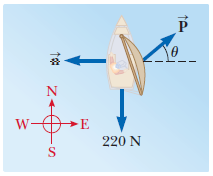# Problem: The figure shows the horizontal forces acting on a sailboat moving north at constant velocity, seen from a point straight above its mast. At the particular speed of the sailboat, the water exerts a 220-N drag force on its hull and θ = 40.0°. For each of the situations (a) and (b) described below, write two component equations representing Newton’s second law. Then solve the equations for P (the force exerted by the wind on the sail) and for n (the force exerted by the water on the keel).(a) Choose the x direction as east and the y direction as north.(b) Now choose the x direction as θ = 40.0° north of east and the y direction as θ = 40.0° west of north.(c) Compare your solutions to parts (a) and (b). Do the results agree? Is one method significantly easier?

###### FREE Expert Solution
92% (165 ratings)
###### Problem Details

The figure shows the horizontal forces acting on a sailboat moving north at constant velocity, seen from a point straight above its mast. At the particular speed of the sailboat, the water exerts a 220-N drag force on its hull and θ = 40.0°. For each of the situations (a) and (b) described below, write two component equations representing Newton’s second law. Then solve the equations for P (the force exerted by the wind on the sail) and for n (the force exerted by the water on the keel).
(a) Choose the x direction as east and the y direction as north.
(b) Now choose the x direction as θ = 40.0° north of east and the y direction as θ = 40.0° west of north.
(c) Compare your solutions to parts (a) and (b). Do the results agree? Is one method significantly easier?Frequently Asked Questions

What scientific concept do you need to know in order to solve this problem?

Our tutors have indicated that to solve this problem you will need to apply the Forces in 2D concept. You can view video lessons to learn Forces in 2D. Or if you need more Forces in 2D practice, you can also practice Forces in 2D practice problems.

How long does this problem take to solve?

Our expert Physics tutor, Julia took 8 minutes and 49 seconds to solve this problem. You can follow their steps in the video explanation above.

What professor is this problem relevant for?

Based on our data, we think this problem is relevant for Professor Monda's class at Hudson Valley Community College.